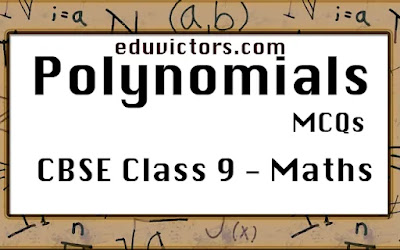CBSE Class 9 - Maths - Polynomials MCQs

Choose the correct option from the choices given in each question.

Q1: Which one of the following is incorrect about polynomials?

(a) A monomial contains one non-zero term.

(b) A polynomial is an algebraic expression.

(c) Exponents of a polynomial can be irrational.

(d) Coefficients of a polynomial can be irrational.

Q2: Which of the following is a constant polynomial?

(a) $x + \frac{1}{x}$

(b) $\sqrt{3}$

(c) $\sqrt{2}x + 7$

(d) $\sqrt{x} - 2$

Q3: What is the degree of zero polynomial?

(a) 0

(b) 1

(c) 2

(d) undefined

Q4: What is the degree of polynomial $p(x) =x^2 -5x^7 - 20 + x^3$?

(a) 2

(b) 3

(c) 7

(d) undefined

Q5: $\sqrt{5}$ is a polynomial of degree?

(a) 5

(b) 0

(c) 1

(d) 2

Q6: Which of the following polynomials has –3 as its zero?

(a) x – 3

(b) x² – 9

(c) x² – 3x

(d) x² + 3

Q7: If $p(x) = 3x^{100} + 2x^{50} - 10$, p(1) = ?

(a) 5

(b) -5

(c) 190

(d) 40

Q8: If $p(x) = x^2 + kx + 6$, find the value of k if p(3) = 0?

(a) –5

(b) 2

(c) 3

(d) –1

Q9: Which one of the following is a correct statement?

(a) A constant polynomial can have many zeroes.

(b) 0 cannot be the zero of a given polynomial.

(c) Every linear polynomial has one and only one zero.

(d) Number of zeros of a polynomial can exceed its degree.

Q10: If p(x) = x + 4 then p(x)+p(-x)=?

(a) 0

(b) 4

(c) 2x

(d) 8

1. (c) Exponents of a polynomial can be irrational.

2. (b) $\sqrt{3}$

3. (d) undefined

4. (c) 7

5. (c) 1

6. (b) x² – 9

7: (b) -5

8: (a) –5

9: (c) Every linear polynomial has one and only one zero.

10: (d) 8